Other forms for proportionsCustom SearchOTHER FORMS FOR PROPORTIONS If four numbers, for example, a, b, c, and d, form a proportion, such asthey also form a proportion according to other arrangements.     Inversion The four selected numbers are in proportion by INVERSION in the form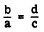The inversion relationship is proved as follows, by first multiplying both members of the original proportion by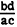: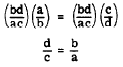Note that the product of the means and the product of the extremes still yield the same equality as in the original proportion. The inversion relationship may be illustrated by the following numerical example:Therefore,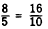Alternation The four selected numbers (a, b, c, and d) are in proportion by ALTERNATION in the following form:To prove the alternation relationship, first multiply both sides of the original proportion by b/ c, as follows: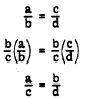The following numerical example illustrates alternation:Therefore,Integrated Publishing, Inc. - A (SDVOSB) Service Disabled Veteran Owned Small Business